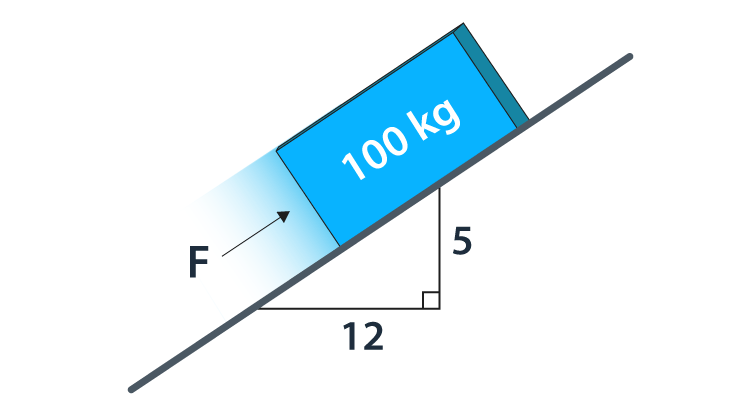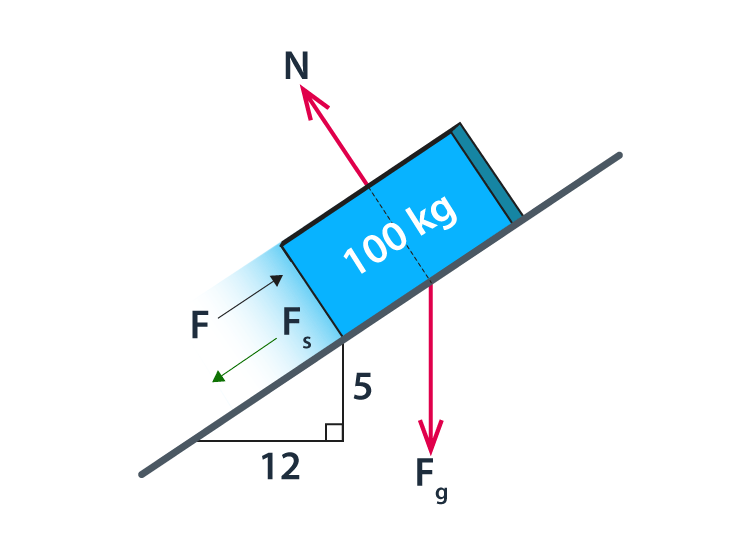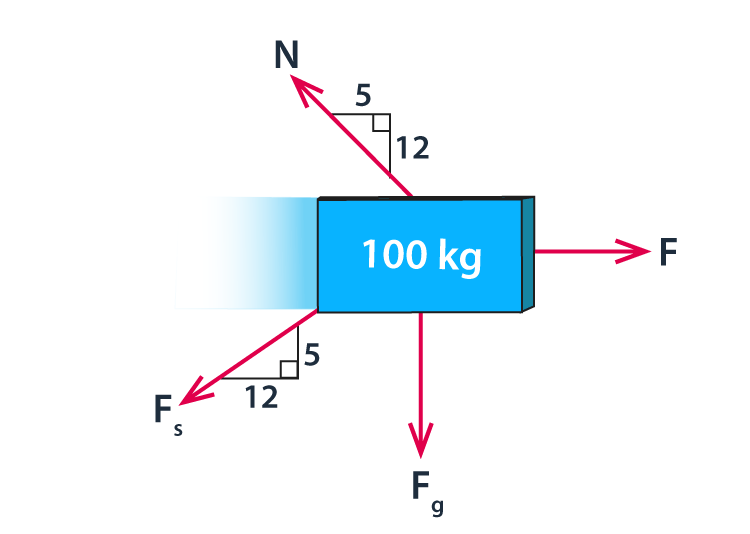# Impending Motion

The impending motion refers to the state of a body when it is on the verge of slipping. In such cases, the static friction has reached its upper limit and is given by the equation

$$\begin{array}{l}F=\mu _{s}N\end{array}$$
The direction of the frictional force is always opposite to the pending relative motion of the surfaces. If the applied force (P) increases then the frictional force (F) also increases, until F<FS(limiting static frictional force). When F = FS, then the body possesses an unstable equilibrium and will move.

To better understand the meaning of impending motion, let us look at the three regions of static to motion transition in the next section.

## Three Regions of Static to Motion Transition

• No Motion – It is the region up till the point of slippage or impending motion. The frictional force in this region is defined by the equations of equilibrium as the system is in equilibrium. When the motion is not impending, F<Fmax.
• Impending Motion – It is the moment when the body is on the verge of slipping. Here, the static friction reaches its upper limit. For a given pair of surfaces in contact, the frictional force is given by the equation
$$\begin{array}{l}F=F_{max}=\mu _sN\end{array}$$
• Motion – In this state, the body starts to move in the direction of the applied force. Here, the frictional force drops to a lower value known as the kinetic friction. The frictional force is given by the equation
$$\begin{array}{l}F=F_{max}=\mu _kN\end{array}$$

The force will drop further with higher velocity.

In the next section, let us solve a problem related to impending motion.

### Finding The Force For Impending Motion

Let us look at the example given below to understand how to find the force required to impend motion in an object.

Example: Determine the force necessary for impending motion up the plane. The coefficient of static friction is 0.2.Solution:

First, let us look at all the forces acting on the body and represent it in the free body diagram.The free body diagram can be represented as follows:The force of gravity acting on the body can be found as follows:

Fg = mg

Substituting the values, we get

Fg = 100 kg × 9.8 m/s2 = 980 N

To maintain equilibrium, the sum of the horizontal forces should be zero and the sum of the vertical forces should be zero.

∑Fx = 0 and ∑Fy = 0

Let us look at the vertical component of forces to calculate the normal force

$$\begin{array}{l}\sum F_{y}=-F_g+N(\frac{12}{13})-F_s\frac{5}{13}=0\end{array}$$

Substituting the value of the force of gravity and the coefficient of static friction in the above equation, we get

$$\begin{array}{l}\sum F_{y}=-980+(\frac{12}{13})N-0.2N\frac{5}{13}=0\end{array}$$
$$\begin{array}{l}\frac{12}{13}N-0.2\frac{5}{13}N=980\end{array}$$
$$\begin{array}{l}N=\frac{980}{\frac{12}{13}-0.2\times\frac{5}{13}}=1158\,N\end{array}$$

The normal force is 1158 N

Now, to find the pushing force let us look at the horizontal forces

$$\begin{array}{l}-N\frac{5}{13}-F_s\frac{12}{13}+F=0\end{array}$$
$$\begin{array}{l}F=N\frac{5}{13}+\mu N\frac{12}{13}\end{array}$$
$$\begin{array}{l}F=N\frac{5}{13}+\mu N\frac{12}{13}\end{array}$$

Substituting the values we get,

$$\begin{array}{l}F=1158\frac{5}{13}+(0.2\times 1158)\frac{12}{13}=659\,N\end{array}$$

Hence, the force required for impending motion is F = 659 N.

## Frequently Asked Questions – FAQs

Q1

### Describe impending motion.

When an object is just about to slip from any surface then the state of the object is known as impending motion. When the static friction reaches its maximum limit then the impending motion occurs.
Q2

### What is kinetic friction?

Kinetic friction is defined as a force that acts between moving surfaces. A body moving on the surface experiences a force in the opposite direction of its movement. The magnitude of the force will depend on the coefficient of kinetic friction between the two materials.
Q3

### Describe maximum static friction.

Maximum static friction is the upper limit of the frictional force which keeps an object in a rest position. When the object reaches the maximum limit of static friction it starts to move in the direction of applied force.
Q4

### How does friction produce heat?

When surfaces in contact move relative to each other, the friction between the two surfaces convert kinetic energy into heat.
Q5

### How is friction useful?

Friction helps us in walking. For example, the friction between the shoes and the floor stops us from slipping. Similarly, the friction between the car and the road prevents the car from sliding.

Stay tuned to BYJU’S to learn more about static friction and its laws with the help of interactive video lessons.

Test your knowledge on Impending motion